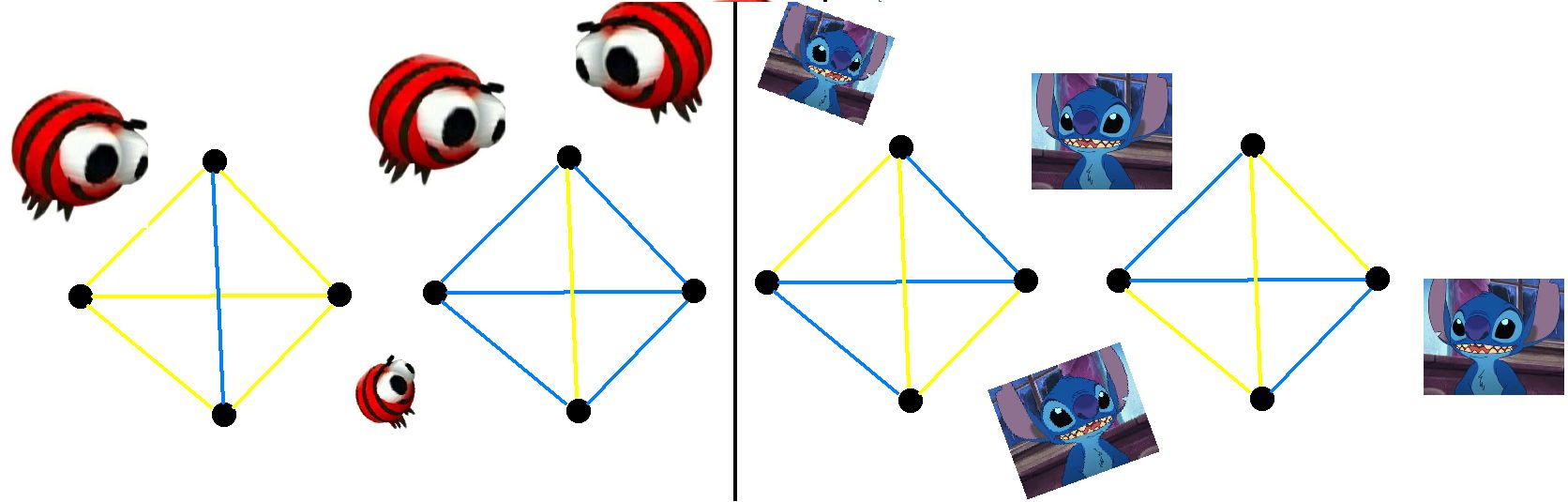시간 제한메모리 제한제출정답맞은 사람정답 비율
3 초 512 MB0000.000%

## 문제

You are given a complete undirected graph on $n$ vertices, each edge is colored blue or yellow. Anton likes a subgraph on $4$ vertices if, among its $6$ edges, $5$ edges have one color, and the $6$-th edge has another color. Yahor likes a subgraph on $4$ vertices if $3$ of its edges are yellow, $3$ are blue, and no $3$ vertices form a triangle with edges of the same color.

On the image below, on the left, you can see examples of graphs Anton likes. On the right, there are examples of graphs Yahor likes.Let $A$ be the number of subgraphs Anton likes, and $Y$ be the number of subgraphs Yahor likes. They want to know who likes more subgraphs. To help them, find the value $Y - A$.

## 입력

The first line of the input contains a single integer $n$ ($4 \le n \le 2000$), the number of vertices in the graph.

The $i$-th of the next $i$ lines contains a string $s_i$ of length $n$.

It is guaranteed that:

• For every $i$ from $1$ to $n$, the $i$-th character of $s_i$ is '-'
• For every $i \neq j$, the $j$-th character of $s_i$ is either 'Y' or 'B', where' Y' shows that the edge between vertices $i$ and $j$ is yellow, and 'B' shows that it is blue
• For every $i \neq j$, the $j$-th character of $s_i$ is equal to the $i$-th character of $s_j$

## 출력

Output a single integer: the value $Y - A$.

## 예제 입력 1

5
-YBYB
Y-BBB
BB-BY
YBB-Y
BBYY-


## 예제 출력 1

2


## 예제 입력 2

6
-YYYYY
Y-YYBB
YY-YYY
YYY-YB
YBYY-Y
YBYBY-


## 예제 출력 2

-6